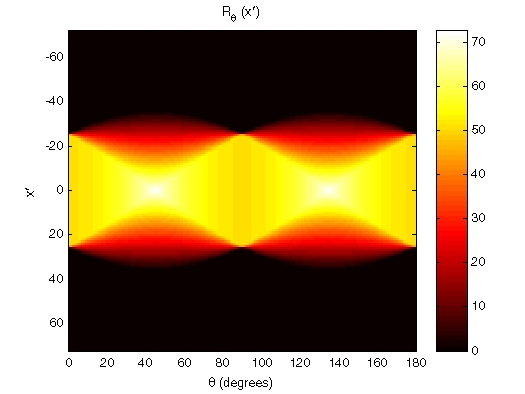Image Processing Toolbox User's GuideViewing the Radon Transform as an Image

The Radon transform for a large number of angles is often displayed as an image. In this example, the Radon transform for the square image is computed at angles from 0° to 180°, in 1° increments.

• theta = 0:180;
imagesc(theta,xp,R);
title('R_{\theta} (X\prime)');
xlabel('\theta (degrees)');
ylabel('X\prime');
set(gca,'XTick',0:20:180);
colormap(hot);
colorbarPlotting the Radon Transform Using the Radon Transform to Detect Lines